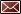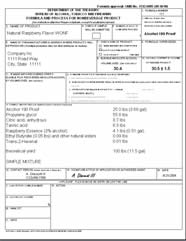Get Email Updates Back to Nonbeverage Products Laboratory page

# Drawback Tutorial

Example formulas

Simple Mixture 2 (including discussion about yield)

This simple mixture is a bit more complex. There are two ingredients that contribute ethanol to this formula.  The 190 proof ethanol is eligible for drawback and will be used to calculate item 9The Raspberry Essence also contains ethanol but it is ineligible for drawback.  However, it must be included in the calculation of item 10.Step 1. Item 9 calculation – as in the previous example, first you need to convert pounds to gallons: 25.0 pounds ÷ 6.7943 pounds/gallon = 3.68 gallons next, multiply 3.68 gallons by the % ethanol to get the volume of absolute alcohol: 3.7 gallons × 0.95 = 3.50 gallons of absolute ethanol the last step is to divide by the yield 3.50 gallons of absolute ethanol ÷ 11.5 gallons = 0.304 or 30.4% for item 9. Step 2. Item 10 calculation – The ethanol from the Raspberry Essence must be added to the ethanol that was calculated for item 9. Click on the image to enlarge

The density of the Raspberry Essence is used to convert the weight to volume. You need only to multiply the volume of the Raspberry Essence by the volume percent ethanol of 3%.

0.51 gallons × 0.030 = 0.015 gallons of absolute ethanol

Add the gallons of absolute ethanol from (1) and (2) together:

0.015 + 3.50 = 3.515 gallons of absolute ethanol

divide this by the yield to get the ethanol content for item 10:

3.535 gallons of absolute ethanol ÷ 11.5 gallons = 0.306 or 30.6%

As in the previous example, the tolerance for item 10 is set according to the guidelines established in the tolerance table for a simple mixture.

Filtered products - The calculation of item 10 would not change.  However, item 9 would be calculated using the final (actual) yield.

In general, the yield of a filtered product is lower than that of a simple mixture (simple mixtures = 100% yield).  We refer to a 100% yield as the theoretical yield because it assumes that none of the product is lost during processing.

For simple mixtures it is easy to see why we can use a 100% yield (there are no processing steps to significantly affect the final yield). However, filtrations expose the product to more processing steps and, as a result, increased probability of product loss.

A formula for a filtered product (that is submitted to us) should include both theoretical and final yields.

So why do we use the final yield to calculate item 9?

This is important because it is not simply the ethanol in the final product that is calculated, it is the ethanol used in the manufacturing process.  This is especially important for products where some or all of the ethanol was lost due to processing.

Why do we use the theoretical yield to calculate item 10?

When filtration is the only other processing step, the ethanol content in the final product will be determined by the proportioning of miscible ingredients during mixing (even though there will be a loss of product to the filtration step).

Since filtration is not a selective process for dissolved components we would not expect the ethanol level to change.